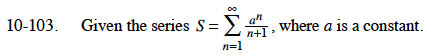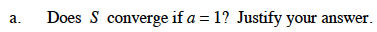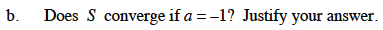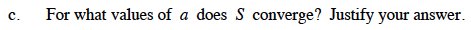### Home > CALC > Chapter 10 > Lesson 10.2.2 > Problem10-103

10-103.
1. Given the series, where a is a constant. Homework Help ✎

3. For what values of a does S converge? Justify your answer.This is a varitation of the harmonic series.This is an alternating series.$\text{Using the Ratio Test: }\lim_{n\to\infty}\Big|\frac{a^{n+1}/(n+2)}{a^n/(n+1)}\Big|<1$